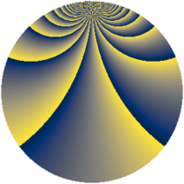# Properties

 Label 1805.2.qLevel $1805$ Weight $2$ Character orbit 1805.q Rep. character $\chi_{1805}(96,\cdot)$ Character field $\Q(\zeta_{19})$ Dimension $2304$ Sturm bound $380$

# Related objects

## Defining parameters

 Level: $$N$$ $$=$$ $$1805 = 5 \cdot 19^{2}$$ Weight: $$k$$ $$=$$ $$2$$ Character orbit: $$[\chi]$$ $$=$$ 1805.q (of order $$19$$ and degree $$18$$) Character conductor: $$\operatorname{cond}(\chi)$$ $$=$$ $$361$$ Character field: $$\Q(\zeta_{19})$$ Sturm bound: $$380$$

## Dimensions

The following table gives the dimensions of various subspaces of $$M_{2}(1805, [\chi])$$.

Total New Old
Modular forms 3456 2304 1152
Cusp forms 3384 2304 1080
Eisenstein series 72 0 72

## Trace form

 $$2304 q - 2 q^{2} + 30 q^{3} - 136 q^{4} - 8 q^{6} - 12 q^{7} - 6 q^{8} - 30 q^{9} + O(q^{10})$$ $$2304 q - 2 q^{2} + 30 q^{3} - 136 q^{4} - 8 q^{6} - 12 q^{7} - 6 q^{8} - 30 q^{9} - 6 q^{10} - 8 q^{11} - 24 q^{12} - 24 q^{13} + 26 q^{14} - 4 q^{15} - 152 q^{16} + 90 q^{17} - 10 q^{18} - 20 q^{19} - 16 q^{21} + 52 q^{22} - 12 q^{23} - 24 q^{24} - 128 q^{25} + 158 q^{26} - 6 q^{27} - 60 q^{28} - 24 q^{29} - 40 q^{31} - 54 q^{32} - 60 q^{33} - 68 q^{34} - 4 q^{35} - 232 q^{36} - 52 q^{37} - 16 q^{38} - 36 q^{39} - 30 q^{40} - 56 q^{41} - 100 q^{42} + 260 q^{43} - 88 q^{44} - 8 q^{45} - 46 q^{46} + 90 q^{47} + 246 q^{48} - 56 q^{49} - 2 q^{50} + 36 q^{51} - 120 q^{52} - 60 q^{53} + 736 q^{54} + 370 q^{56} - 76 q^{57} - 84 q^{58} - 40 q^{59} + 26 q^{60} - 72 q^{61} - 132 q^{62} + 110 q^{63} - 220 q^{64} - 20 q^{65} - 120 q^{66} - 52 q^{67} - 116 q^{68} + 40 q^{69} - 28 q^{70} - 48 q^{71} - 130 q^{72} - 96 q^{73} + 70 q^{74} - 8 q^{75} + 12 q^{76} - 64 q^{77} + 364 q^{78} - 64 q^{79} + 136 q^{80} + 18 q^{81} + 48 q^{82} - 6 q^{83} - 200 q^{84} - 16 q^{85} + 310 q^{86} + 154 q^{87} - 164 q^{88} + 18 q^{89} + 264 q^{90} - 58 q^{91} - 136 q^{92} - 56 q^{93} + 212 q^{94} - 12 q^{95} - 162 q^{96} - 34 q^{97} - 4 q^{98} - 120 q^{99} + O(q^{100})$$

## Decomposition of $$S_{2}^{\mathrm{new}}(1805, [\chi])$$ into newform subspaces

The newforms in this space have not yet been added to the LMFDB.

## Decomposition of $$S_{2}^{\mathrm{old}}(1805, [\chi])$$ into lower level spaces

$$S_{2}^{\mathrm{old}}(1805, [\chi]) \cong$$ $$S_{2}^{\mathrm{new}}(361, [\chi])$$$$^{\oplus 2}$$